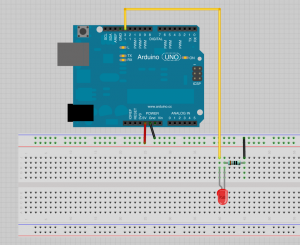# What is electricity?

### Ohm’s Law

Ohm’s law is an equation that can be used to calculate the values of the different properties in an electronic circuit.

• R (resistance) = V (voltage) / I (current)
• V=R * I
• I=V / R

This video from Make explains the basics quite nicely:

Here is a good definition of the basic concepts and components you will run into by Tom Igoe http://www.tigoe.com/pcomp/code/circuits/understanding-electricity/

# Arduino

Download the Arduino software and follow the instructions on the Arduino website if you have problems setting things up. The Arduino boards that you have are Arduino Unos and do not require any driver installation for Mac or Linux.

Getting Started With Arduino from the Arduino website

### Arduino Code Structure

The Arduino software is used to program the Arduino. You will notice that it is very similar to Processing. The code structure is also very similar. All Arduino sketches need to have the following functions.

• setup()
• Runs once when the board starts or is reset
• You use the setup() for things that you usually only need to do once (like opening the serial port, defining pin modes etc.)
• loop()
• Runs continuously as long as the board has power

Our first program is the one that already comes with your Arduino. You will see that the little LED on the board marked ‘L’ starts blinking when you power the board. Let’s connect an external LED to see it a little bit better. There is a good tutorial on the Arduino website for this basic example.The code:

```/*
Turns on an LED on for one second, then off for one second, repeatedly.

This example code is in the public domain.
*/

// Pin 13 has an LED connected on most Arduino boards.
// give it a name:
int led = 13;

// the setup routine runs once when you press reset:
void setup() {
// initialize the digital pin as an output.
pinMode(led, OUTPUT);
}

// the loop routine runs over and over again forever:
void loop() {
digitalWrite(led, HIGH);   // turn the LED on (HIGH is the voltage level)
delay(1000);               // wait for a second
digitalWrite(led, LOW);    // turn the LED off by making the voltage LOW
delay(1000);               // wait for a second
}```